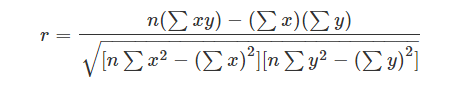# Coefficient of Determination

In Statistical Analysis, the coefficient of determination method is used to predict and explain the future outcomes of a model. This method is also known as R squared. This method also acts like a guideline which helps in measuring the model’s accuracy. In this article, let us discuss the definition, formula, and properties of the coefficient of determination in detail.

## Coefficient of Determination Definition

The coefficient of determination or R squared method is the proportion of the variance in the dependent variable that is predicted from the independent variable. It indicates the level of variation in the given data set.

• The coefficient of determination is the square of the correlation(r), thus it ranges from 0 to 1.
• With linear regression, the coefficient of determination is equal to the square of the correlation between the x and y variables.
• If R2 is equal to 0, then the dependent variable cannot be predicted from the independent variable.
• If R2 is equal to 1, then the dependent variable can be predicted from the independent variable without any error.
• If R2 is between 0 and 1, then it indicates the extent that the dependent variable can be predictable. If Rof 0.10 means, it is 10 percent of the variance in the y variable is predicted from the x variable. If 0.20 means, 20 percent of the variance in the y variable is predicted from the x variable, and so on.

The value of R2 shows whether the model would be a good fit for the given data set. In the context of analysis, for any given per cent of the variation, it(good fit) would be different. For instance, in a few fields like rocket science, R2 is expected to be nearer to 100 %. But R2 = 0(minimum theoretical value), which might not be true as R2 is always greater than 0( by Linear Regression).

The value of R2 increases after adding a new variable predictor. Note that it might not be associated with the result or outcome. The R2 which was adjusted will include the same information as the original one. The number of predictor variables in the model gets penalized. When in a multiple linear regression model, new predictors are added, it would increase R2. Only an increase in R2 which is greater than the expected(chance alone), will increase the adjusted R2.

Following is the Regression line equation

p’ = aq + r

Where ‘p’ is the predicted function value of q. So, the method of checking how good the least-squares equation p̂ = aq + r will make a prediction of how p will be made.

## Coefficient of Determination Formula

We can give the formula to find the coefficient of determination in two ways; one using correlation coefficient and the other one with sum of squares.

Formula 1:

As we know the formula of correlation coefficient is,Where

n = Total number of observations

Σx = Total of the First Variable Value

Σy = Total of the Second Variable Value

Σxy = Sum of the Product of first & Second Value

Σx2 = Sum of the Squares of the First Value

Σy2 = Sum of the Squares of the Second Value

Thus, the coefficient of of determination = (correlation coefficient)2 = r2

Formula 2:

The formula of coefficient of determination is given by:

Where,

R2 = Coefficient of Determination

RSS = Residuals sum of squares

TSS = Total sum of squares

### Properties of Coefficient of Determination

• It helps to get the ratio of how a variable which can be predicted from the other one, varies.
• If we want to check how clear it is to make predictions from the data given, we can determine the same by this measurement.
• It helps to find Explained variation / Total Variation
• It also lets us know the strength of the association(linear) between the variables.
• If the value of r2 gets close to 1, The values of y become close to the regression line and similarly if it goes close to 0, the values get away from the regression line.
• It helps in determining the strength of association between different variables.

### Steps to Find the Coefficient of Determination

1. Find r, Correlation Coefficient
2. Square ‘r’.
3. Change the above value to a percentage.

## Frequently Asked Questions – FAQs

Q1

### How is R^2 calculated?

The value of R^2 is calculated using the below formula.
Here,
RSS = Residuals sum of squares
TSS = Total sum of squares
Q2

### How is the coefficient of determination calculated?

Using the correlation coefficient formula, the coefficient of determination can be calculated in three steps.
Step 1: Find r, the correlation coefficient
Step 2: Square the value of ‘r’
Step 3: Change the obtained value to a percentage
Q3

### What is a good coefficient of determination?

Generally, the coefficient of determination with about 70% is considered good. Also, we can say that 50% of this is considered a moderate fit for the given model.
Q4

### Is the coefficient of determination the same as R^2?

Yes, the coefficient of determination is denoted by R^2.
Q5

### What does R^2 tell us?

R^2 or R-squared is a statistical measure of how close the data are to the fitted regression line. It is also called the coefficient of determination.